# 2.2 Graphs of linear functions  (Page 10/15)

 Page 10 / 15

$\begin{array}{c}3y+x=12\\ -y=8x+1\end{array}$

neither parallel or perpendicular

$\begin{array}{c}3y+4x=12\\ -6y=8x+1\end{array}$

$\begin{array}{c}6x-9y=10\\ 3x+2y=1\end{array}$

perpendicular

$\begin{array}{c}y=\frac{2}{3}x+1\\ 3x+2y=1\end{array}$

$\begin{array}{c}y=\frac{3}{4}x+1\\ -3x+4y=1\end{array}$

parallel

For the following exercises, find the x - and y- intercepts of each equation

$f\left(x\right)=-x+2$

$g\left(x\right)=2x+4$

;

$h\left(x\right)=3x-5$

$k\left(x\right)=-5x+1$

;

$-2x+5y=20$

$7x+2y=56$

;

For the following exercises, use the descriptions of each pair of lines given below to find the slopes of Line 1 and Line 2. Is each pair of lines parallel, perpendicular, or neither?

• Line 1: Passes through $\left(0,6\right)$ and $\left(3,-24\right)$
• Line 2: Passes through $\left(-1,19\right)$ and $\left(8,-71\right)$
• Line 1: Passes through $\left(-8,-55\right)$ and $\left(10,\text{\hspace{0.17em}}89\right)$
• Line 2: Passes through $\left(9,-44\right)$ and $\left(4,-14\right)$

• Line 1: Passes through $\left(2,3\right)$ and $\left(4,-1\right)$
• Line 2: Passes through $\left(6,3\right)$ and $\left(8,5\right)$
• Line 1: Passes through $\left(1,7\right)$ and $\left(5,5\right)$
• Line 2: Passes through $\left(-1,-3\right)$ and $\left(1,1\right)$

• Line 1: Passes through $\left(0,5\right)$ and $\left(3,3\right)$
• Line 2: Passes through $\left(1,-5\right)$ and $\left(3,-2\right)$
• Line 1: Passes through $\left(2,5\right)$ and $\left(5,-1\right)$
• Line 2: Passes through $\left(-3,7\right)$ and $\left(3,-5\right)$

Write an equation for a line parallel to $f\left(x\right)=-5x-3$ and passing through the point

Write an equation for a line parallel to $g\left(x\right)=3x-1$ and passing through the point $\left(4,9\right).$

$g\left(x\right)=3x-3$

Write an equation for a line perpendicular to $h\left(t\right)=-2t+4$ and passing through the point

Write an equation for a line perpendicular to $p\left(t\right)=3t+4$ and passing through the point $\left(3,1\right).$

$p\left(t\right)=-\frac{1}{3}t+2$

Find the point at which the line $f\left(x\right)=-2x-1$ intersects the line $g\left(x\right)=-x.$

Find the point at which the line $f\left(x\right)=2x+5$ intersects the line $g\left(x\right)=-3x-5.$

$\left(-2,1\right)$

Use algebra to find the point at which the line intersects the line $h\left(x\right)=\frac{9}{4}x\text{\hspace{0.17em}}\text{\hspace{0.17em}}+\text{\hspace{0.17em}}\text{\hspace{0.17em}}\frac{73}{10}.$

Use algebra to find the point at which the line $f\left(x\right)=\frac{7}{4}x\text{\hspace{0.17em}}\text{\hspace{0.17em}}+\text{\hspace{0.17em}}\text{\hspace{0.17em}}\frac{457}{60}$ intersects the line $g\left(x\right)=\frac{4}{3}x\text{\hspace{0.17em}}\text{\hspace{0.17em}}+\text{\hspace{0.17em}}\text{\hspace{0.17em}}\frac{31}{5}.$

$\left(-\frac{17}{5},\frac{5}{3}\right)$

## Graphical

For the following exercises, the given linear equation with its graph in [link] .

$f\left(x\right)=-x-1$

$f\left(x\right)=-2x-1$

F

$f\left(x\right)=-\frac{1}{2}x-1$

$f\left(x\right)=2$

C

$f\left(x\right)=2+x$

$f\left(x\right)=3x+2$

A

For the following exercises, sketch a line with the given features.

An x -intercept of and y -intercept of

An x -intercept of and y -intercept of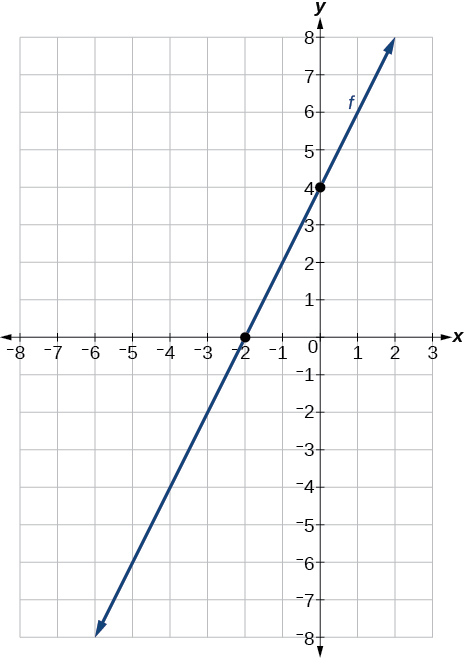A y -intercept of and slope $-\frac{3}{2}$

A y -intercept of and slope $\frac{2}{5}$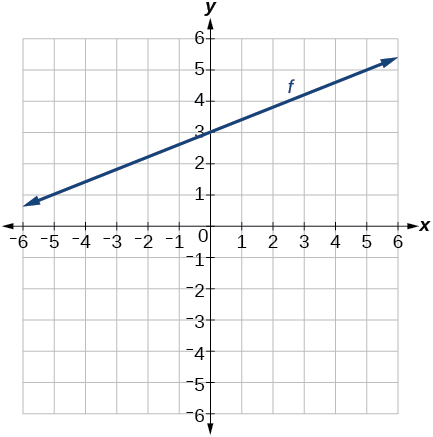Passing through the points and

Passing through the points and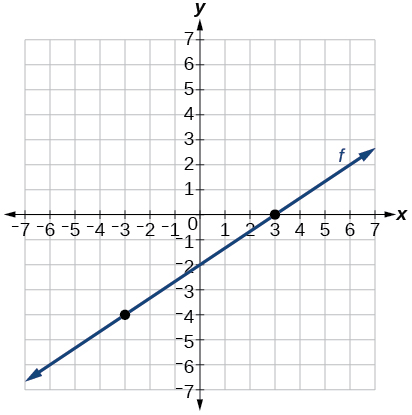For the following exercises, sketch the graph of each equation.

$f\left(x\right)=-2x-1$

$g\left(x\right)=-3x+2$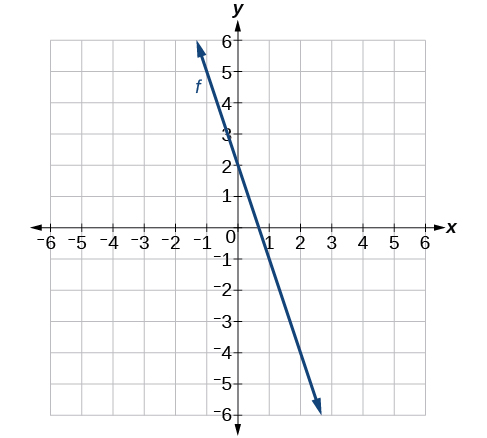$h\left(x\right)=\frac{1}{3}x+2$

$k\left(x\right)=\frac{2}{3}x-3$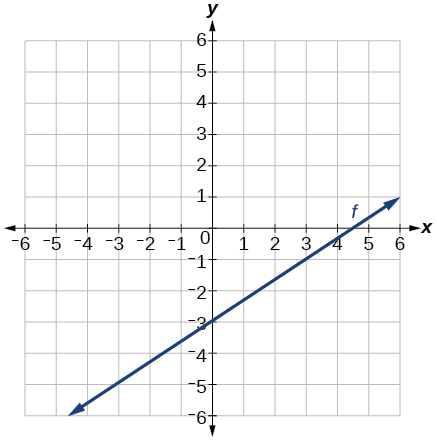$f\left(t\right)=3+2t$

$p\left(t\right)=-2\text{\hspace{0.17em}}+\text{\hspace{0.17em}}3t$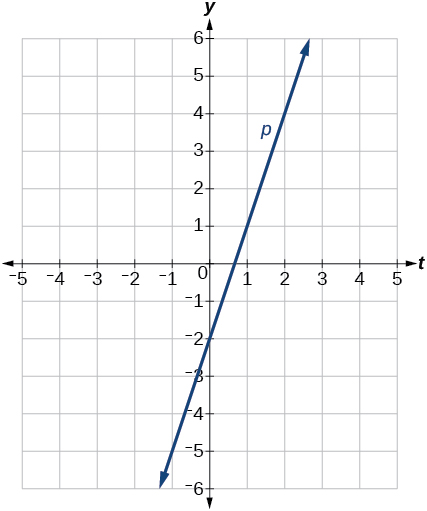$x=3$

$x=-2$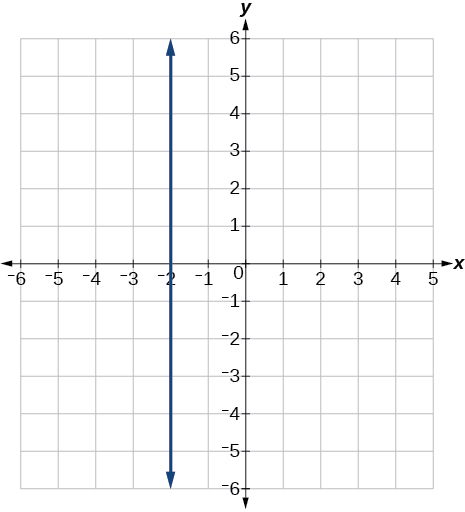$r\left(x\right)=4$

$q\left(x\right)=3$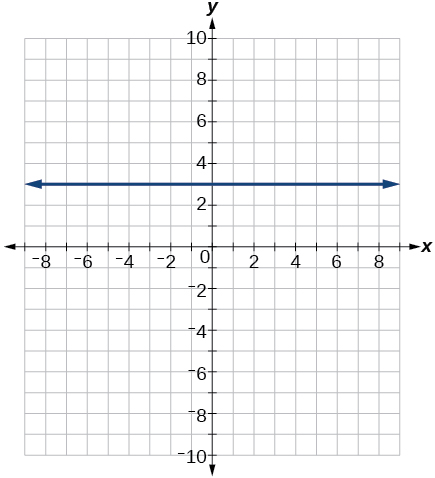$4x=-9y+36$

$\frac{x}{3}-\frac{y}{4}=1$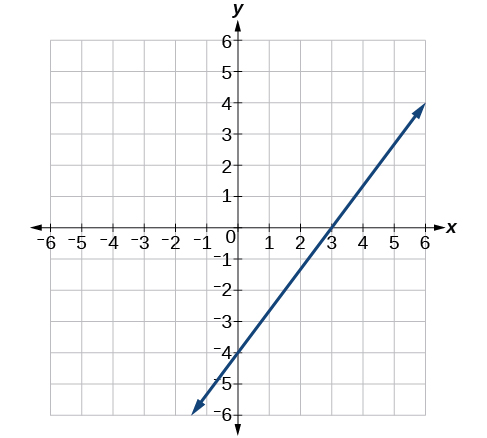$3x-5y=15$

$3x=15$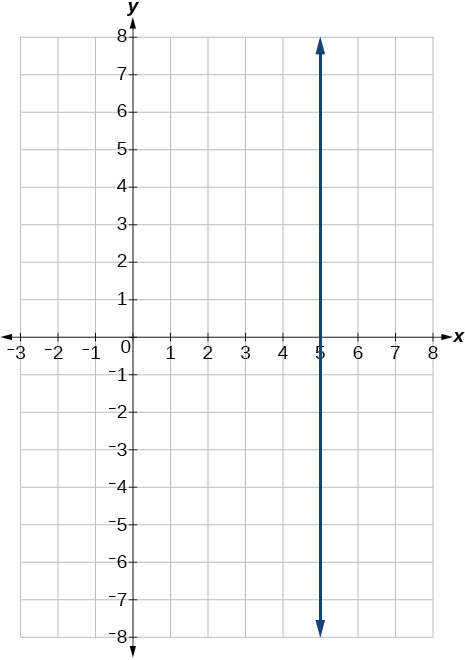$3y=12$

If $g\left(x\right)$ is the transformation of $f\left(x\right)=x$ after a vertical compression by $\frac{3}{4},$ a shift right by 2, and a shift down by 4

1. Write an equation for $g\left(x\right).$
2. What is the slope of this line?
3. Find the y- intercept of this line.

• $g\left(x\right)=0.75x-5.5\text{}$
• 0.75
• $\left(0,-5.5\right)$

If $g\left(x\right)$ is the transformation of $f\left(x\right)=x$ after a vertical compression by $\frac{1}{3},$ a shift left by 1, and a shift up by 3

1. Write an equation for $g\left(x\right).$
2. What is the slope of this line?
3. Find the y- intercept of this line.

For the following exercises,, write the equation of the line shown in the graph.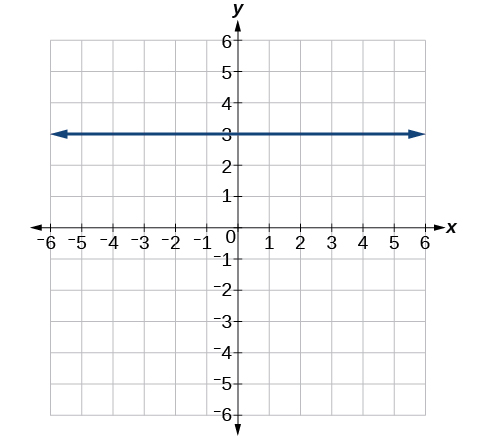$y=\text{3}$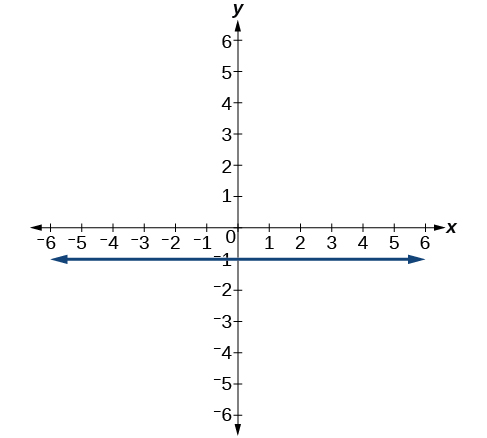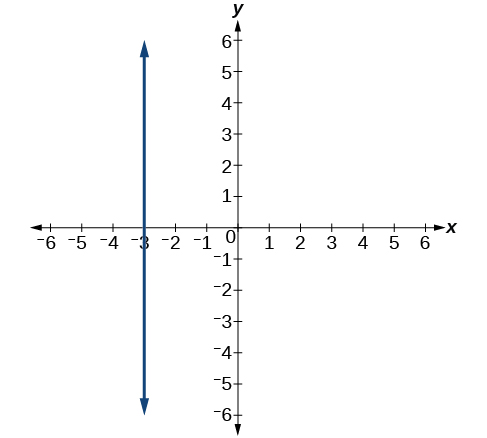$x=-3$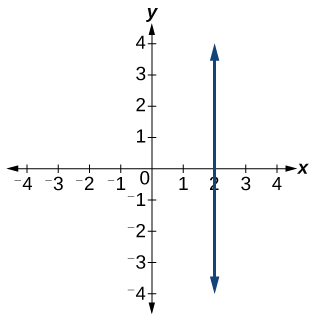For the following exercises, find the point of intersection of each pair of lines if it exists. If it does not exist, indicate that there is no point of intersection.

$\begin{array}{c}y=\frac{3}{4}x+1\\ -3x+4y=12\end{array}$

no point of intersection

$\begin{array}{c}2x-3y=12\\ 5y+x=30\end{array}$

$\begin{array}{c}2x=y-3\\ y+4x=15\end{array}$

$\begin{array}{c}x-2y+2=3\\ x-y=3\end{array}$

$\begin{array}{c}5x+3y=-65\\ x-y=-5\end{array}$

## Extensions

Find the equation of the line parallel to the line $g\left(x\right)=-0.\text{01}x\text{\hspace{0.17em}}\text{+}\text{\hspace{0.17em}}\text{2}\text{.01}$ through the point

Find the equation of the line perpendicular to the line $g\left(x\right)=-0.\text{01}x\text{+2}\text{.01}$ through the point

$y=100x-98$

For the following exercises, use the functions

Find the point of intersection of the lines $f$ and $g.$

Where is $f\left(x\right)$ greater than $g\left(x\right)?$ Where is $g\left(x\right)$ greater than $f\left(x\right)?$

$x<\frac{1999}{201}x>\frac{1999}{201}$

## Real-world applications

A car rental company offers two plans for renting a car.

• Plan A: $30 per day and$0.18 per mile
• Plan B: $50 per day with free unlimited mileage How many miles would you need to drive for plan B to save you money? A cell phone company offers two plans for minutes. • Plan A:$20 per month and $1 for every one hundred texts. • Plan B:$50 per month with free unlimited texts.

How many texts would you need to send per month for plan B to save you money?

Less than 3000 texts

A cell phone company offers two plans for minutes.

• Plan A: $15 per month and$2 for every 300 texts.
• Plan B: $25 per month and$0.50 for every 100 texts.

How many texts would you need to send per month for plan B to save you money?

difference between calculus and pre calculus?
give me an example of a problem so that I can practice answering
x³+y³+z³=42
Robert
dont forget the cube in each variable ;)
Robert
of she solves that, well ... then she has a lot of computational force under her command ....
Walter
what is a function?
I want to learn about the law of exponent
explain this
what is functions?
A mathematical relation such that every input has only one out.
Spiro
yes..it is a relationo of orders pairs of sets one or more input that leads to a exactly one output.
Mubita
Is a rule that assigns to each element X in a set A exactly one element, called F(x), in a set B.
RichieRich
If the plane intersects the cone (either above or below) horizontally, what figure will be created?
can you not take the square root of a negative number
No because a negative times a negative is a positive. No matter what you do you can never multiply the same number by itself and end with a negative
lurverkitten
Actually you can. you get what's called an Imaginary number denoted by i which is represented on the complex plane. The reply above would be correct if we were still confined to the "real" number line.
Liam
Suppose P= {-3,1,3} Q={-3,-2-1} and R= {-2,2,3}.what is the intersection
can I get some pretty basic questions
In what way does set notation relate to function notation
Ama
is precalculus needed to take caculus
It depends on what you already know. Just test yourself with some precalculus questions. If you find them easy, you're good to go.
Spiro
the solution doesn't seem right for this problem
what is the domain of f(x)=x-4/x^2-2x-15 then
x is different from -5&3
Seid
All real x except 5 and - 3
Spiro
***youtu.be/ESxOXfh2Poc
Loree
how to prroved cos⁴x-sin⁴x= cos²x-sin²x are equal
Don't think that you can.
Elliott
By using some imaginary no.
Tanmay
how do you provided cos⁴x-sin⁴x = cos²x-sin²x are equal
What are the question marks for?
ElliottByByBy Frank LevyBy Keyaira BraxtonBy OpenStaxBy Angelica LitoBy Tess ArmstrongBy Steve GibbsBy Brooke DelaneyBy OpenStaxBy OpenStaxBy OpenStax